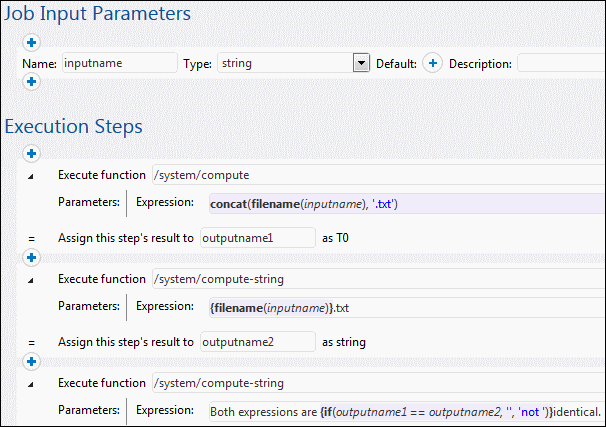# Altova FlowForce Server 2022

## compute-string

Full path: /system/compute-string

Outputs the result of an expression as a string. This step function does essentially the same at the compute function, except that the input format is a string instead of an expression.

## Parameters

Name

Type

Description

Expression

string

The FlowForce Server expression (as string) to be computed.

## Examples

To understand the difference between the /system/compute/ and /system/compute-string functions, consider the following example:In the job illustrated above, there are three execution steps.

The first step calls the /system/compute/ function. Notice that no curly braces were used. The entire field stores an expression (as suggested by the background color), so curly braces are implied. The expression concatenates two values and produces a string depending on the job input parameter. For example, if the input parameter is "c:\temp\invoices.txt", the step will return the string value "invoices.txt" (declared as outputname1).

The second step calls the /system/compute-string function. This function processes a string which contains an embedded FlowForce expression. Here, curly braces are used to delimit the expression from the rest of the string. Notice that the embedded expression has a background color other than the rest of the string. Although a different technique was used, the step result (outputname2) is the same as outputname1.

Finally, the third step calls the /system/compute-string function again, in order to compare the outputname1 with outpuname2. If both values are identical, the result will be the string value "Both expression are identical". Otherwise, the result will be "Both expressions are not identical".